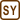## Clock Drift at Higher Temperature

### Task number: 1806

What is the change of an oscillation period of a brass pendulum, which is at 10 °C equal to 1 s, if we increase the ambient temperature to 25 °C?

How much time would a clock with such a pendulum lose per day?

• #### Hint

Realize what happens with the length of a brass pendulum, if we increase the temperature. What impact does it have on the oscillation period of the pendulum?

• #### Analysis

At the temperature of 10 °C, the oscillation period of a brass pendulum is 1 s. At a higher temperature, the length of the pendulum increases due to thermal expansion. The oscillation period of the pendulum increases, because it depends on its length.

• #### Given values

 t1 = 10 °C initial temperature t2 = 25 °C increased temperature Δτ = ? change of oscillation period of the pendulum at higher temperature τt = ? total clock drift per one day

From The Handbook of Chemistry and Physics:

 α = 20·10−6 °C−1 coefficient of linear thermal expansion of brass
• #### Solution

The oscillation period τ1 of a pendulum at initial temperature t1 is described as follows:

$\tau_1=2\pi\sqrt{\frac{l_1}{g}}\,,$

where l1 is the initial length of the pendulum and g is the acceleration of gravity. The acceleration of gravity does not depend on temperature; however, the length of the pendulum increases with increasing temperature linearly so that at temperature t2 it can be calculated according to the relation:

$l_2=l_1\left(1+\alpha\,\mathrm{\Delta}t\right)=l_1\left[1+\alpha\left(t_2-t_1\right)\right]\,.$

This means that the oscillation period increases to τ2 after we increase the temperature.

$\tau_2=2\pi\sqrt{\frac{l_2}{g}}=2\pi\sqrt{\frac{l_1\left[1+\alpha\left(t_2-t_1\right)\right]}{g}}=2\pi\sqrt{\frac{l_1}{g}} \,\sqrt{1+\alpha\left(t_2-t_1\right)}$ $\tau_2=\tau_1\sqrt{1+\alpha\left(t_2-t_1\right)}\,.$

Therefore the clock loses time Δτ every second:

$\mathrm{\Delta}\tau=\tau_2-\tau_1.$

We multiply the calculated time lost in one swing Δτ by the number of seconds in one day and thus obtain the time lost per day. For the total time Δτt lost we get

$\mathrm{\Delta}\tau_t=24{\cdot}60\cdot60\cdot\mathrm{\Delta}\tau=86{,}400\cdot\mathrm{\Delta}\tau.$

Numerical solution

$\tau_2=\tau_1\sqrt{1+\alpha\left(t_2-t_1\right)}=1\sqrt{1+19\cdot{10^{-6}}\left(25-10\right)}\dot\,\mathrm{s}=1.00014\,\mathrm{s}$ $\mathrm{\Delta}\tau=\tau_2-\tau_1=\left(1.00014-1\right)\,\mathrm{s}=0.00014\,\mathrm{s}$ $\mathrm{\Delta}\tau_t=86{,}400\cdot\mathrm{\Delta}\tau=86{,}400\cdot{0.00014}\,\mathrm{s}\dot=12\,\mathrm{s}$
• #### Answer

After increasing the ambient temperature, the oscillation period increases to a value of about 1.00014 s, which gives a total time lost per day of about 12 s (i.e. one day measured by this clock lasts about 12 seconds longer).

• #### Note

Today, we need to measure time very accurately (e.g. because of GPS and other devices). As you have noticed, the precision of pendulum clock depends very strongly on temperature and does not reach required accuracy. For better time measurement, atomic clocks are used today, since they lose only 1 second per 15 million years. Optical clock are even more accurate: they lose one second per a few tens of billions of years!